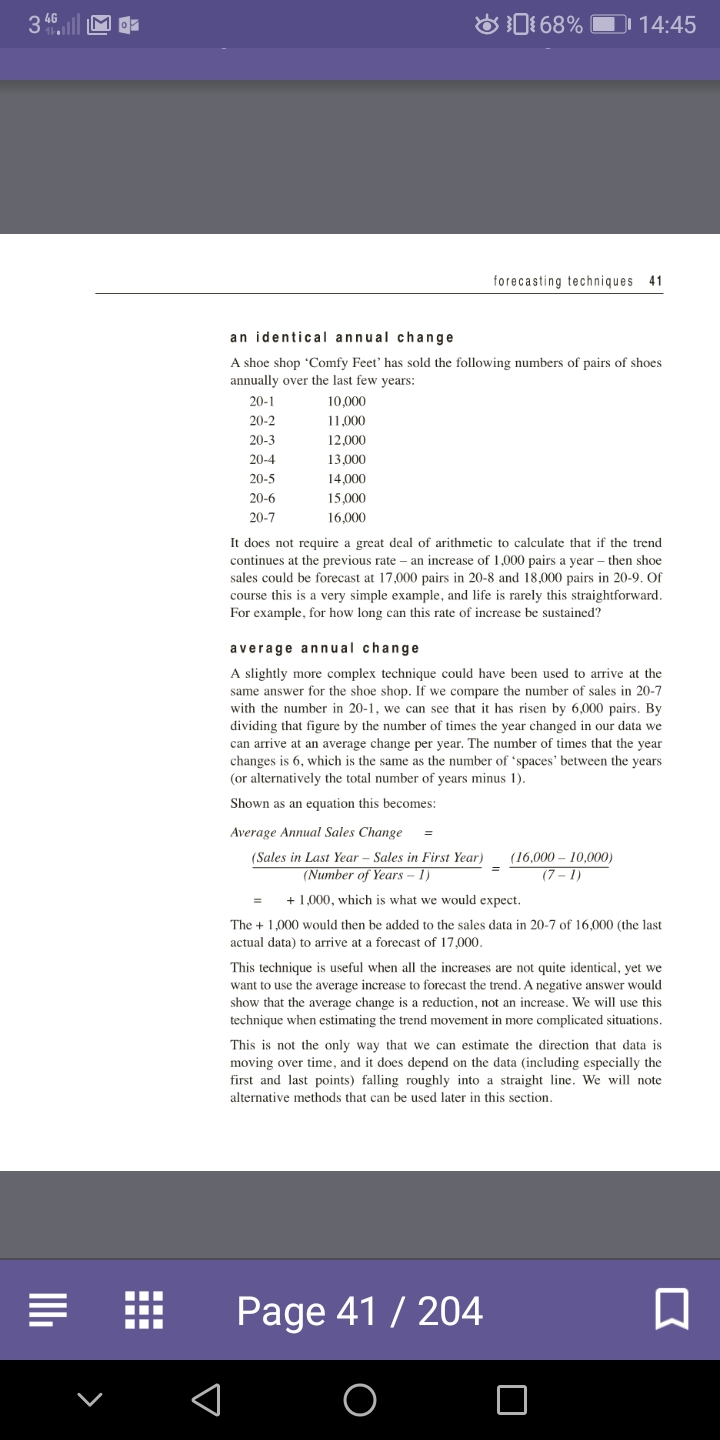# Forecasting

Hi,

I’m hoping someone can explain the attached for me I cannot work out how they got the figures for the trend in 2016.

Thanks
Sophie

• Trend increase is 8, you can use formula 68-44/3, but anyway is obvious
So 68+8=76 and 76+8=84 and so on for the 2016 trend

AAT Level 2. 2018 Distinction
AAT Level 3. 2018 Distinction
AAT Level 4. 2019 FSLC 100% MABU 93% MADC 82% PDSY 80%
• dumutroba said:

Trend increase is 8, you can use formula 68-44/3, but anyway is obvious
So 68+8=76 and 76+8=84 and so on for the 2016 trend

Thanks Dumutroba, without sound silly, how would i know the increase is 8 as this wasn't included in the quesion only on the answers? also the formula you gave why is it divide by 3? and is it also the same formula
• Have a look at this
AAT Level 2. 2018 Distinction
AAT Level 3. 2018 Distinction
AAT Level 4. 2019 FSLC 100% MABU 93% MADC 82% PDSY 80%
•